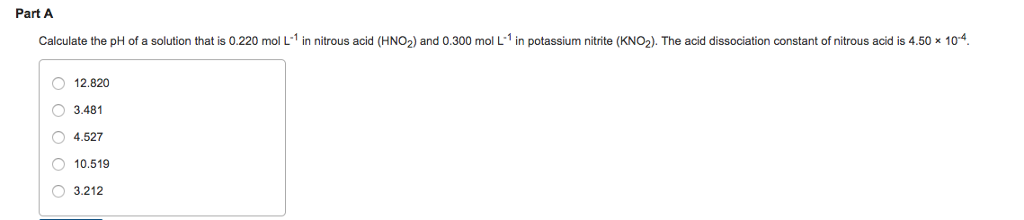# Problem: Calculate the pH of a solution that is 0.220 mol L-1 in nitrous acid (HNO2) and 0.300 mol L-1 in potassium nitrite (KNO2). The acid dissociation constant of nitrous acid is 4.50x10-4. 12.820, 3.481, 4.527, 10.519, 3.212

###### FREE Expert Solution
84% (398 ratings)###### Problem Details

Calculate the pH of a solution that is 0.220 mol L-1 in nitrous acid (HNO2) and 0.300 mol L-1 in potassium nitrite (KNO2).

The acid dissociation constant of nitrous acid is 4.50x10-4

12.820, 3.481, 4.527, 10.519, 3.212